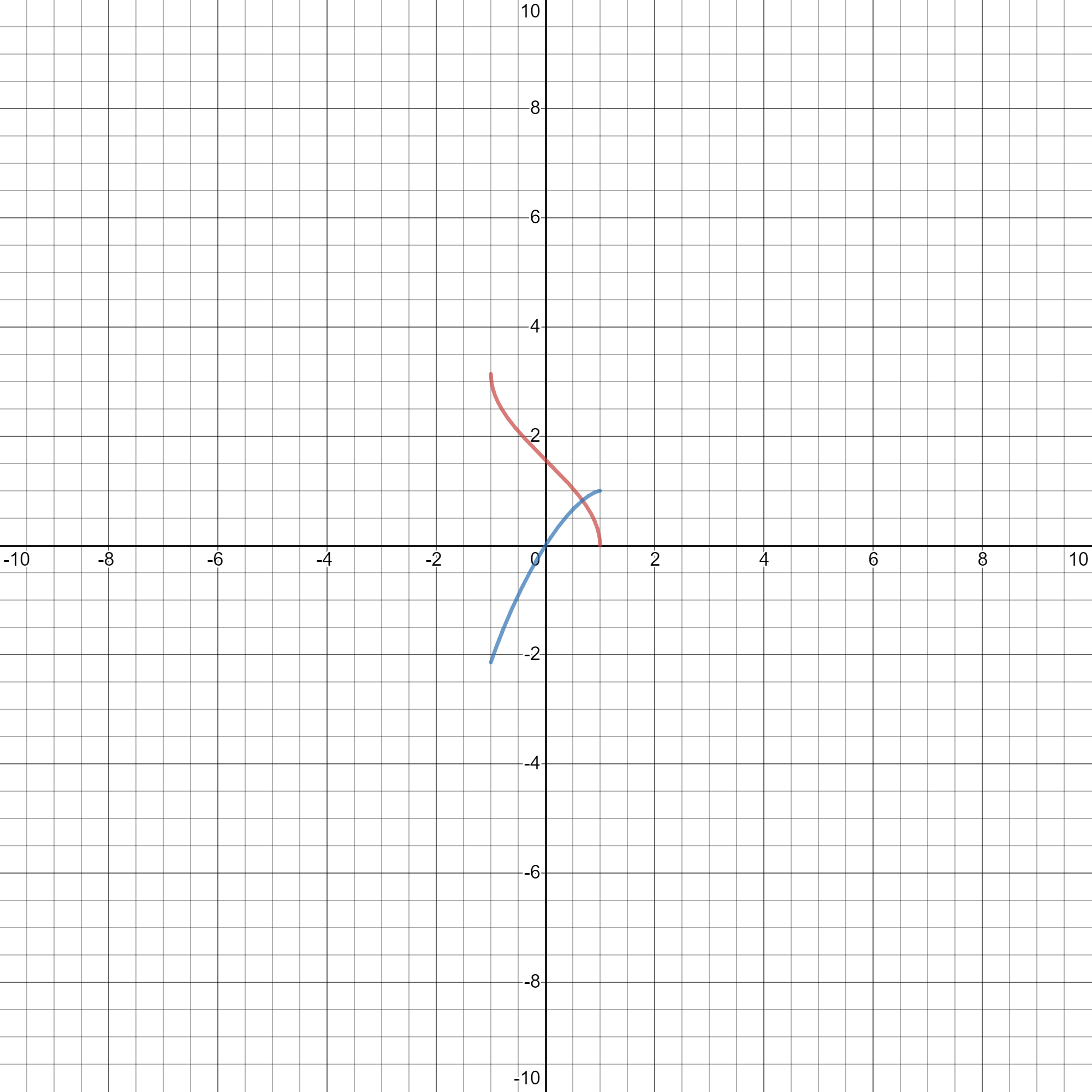# Category: Integration## Integral of arccos(x)

In this post I am going to show you how to integrate the inverse function of cos(x), acos(x), step-by-step. But first, let’s clear a few things. What is arccos(x)? Arccosine… Read more Integral of arccos(x)## Integral of ln(x)

In this post we will find the antiderivative of ln(x) step-by-step. This is one of those integrals you solve once and never forget. Let’s start! What is ln(x)? ln is… Read more Integral of ln(x)## Integral of cot(x)

In this post you’ll learn how to integrate the trigonometric function cot(x). But first: What is cot(x)? Maybe you already know it, but this is going to be really useful.… Read more Integral of cot(x)## Integral of tan(x)

Maybe you know that the answer is ln(sec(x)), but do you know why? Here you’ll find the procedure with explanation! First of all, let’s write the integral: We know that… Read more Integral of tan(x)

## How to solve definite integrals

Indefinite integrals are used to find the formula for the area under a curve of function f(x), whereas definite integrals allow you to calculate the value of the area. It’s like the area of a rectangle, b*h: this is the formula, and in order to find the value you have to plug in the values of b and h. The same is for definite integrals. A definite integral is generally written like this: where a and b are called respectively lower and upper bounds. Also, As you can see, if… Read more How to solve definite integrals

## Integral of S(x) and C(x)

Here I talked about special functions, and at the end of the post I said I would explain how to integrate these special functions. So here I am, showing you how to integrate the functions S(x) and C(x). If you gave it a try, let me know in the comments if you used this approach! Integral of S(x) Like we did for the previous integrals, we are going to use integration by parts, as it is the only way to go. Now we can use u-substitution to solve the integral:… Read more Integral of S(x) and C(x)

{{#pages}} {{/pages}}
%%footer%%